﻿ Synthetic Floating Inductors realized with only two Current Feedback Op-amps

## Synthetic Floating Inductors realized with only two Current Feedback Op-amps

D. R. Bhaskar1, R. Senani2,1Department of Electronics and Communication Engineering, Jamia Millia Islamia, New Delhi, India

2Devision of Electronics and Communication Engineering, Netaji Subhas Institute of Technology, New Delhi, India

### Abstract

Two floating inductance (FI) circuits are presented which employ a canonical number of passive components (namely, only two resistors and a capacitor) as well as canonical number of active elements (only two CFOAs) and realize single-resistance-tunable inductance value, without requiring any component-matching or cancellation constraints. The workability of the proposed circuits and their applications has been confirmed by hardware implementation and SPICE simulations based on AD844-type CFOAs.

• D. R. Bhaskar, R. Senani. Synthetic Floating Inductors realized with only two Current Feedback Op-amps. American Journal of Electrical and Electronic Engineering. Vol. 3, No. 4, 2015, pp 88-92. http://pubs.sciepub.com/ajeee/3/4/1
• Bhaskar, D. R., and R. Senani. "Synthetic Floating Inductors realized with only two Current Feedback Op-amps." American Journal of Electrical and Electronic Engineering 3.4 (2015): 88-92.
• Bhaskar, D. R. , & Senani, R. (2015). Synthetic Floating Inductors realized with only two Current Feedback Op-amps. American Journal of Electrical and Electronic Engineering, 3(4), 88-92.
• Bhaskar, D. R., and R. Senani. "Synthetic Floating Inductors realized with only two Current Feedback Op-amps." American Journal of Electrical and Electronic Engineering 3, no. 4 (2015): 88-92.

 Import into BibTeX Import into EndNote Import into RefMan Import into RefWorks

1
Prev Next

### 1. Introduction

The simulation of grounded inductance (GI) and floating inductance (FI) has continued to remain an important and popular area of analog circuits research due to their applications in linear (active filters and oscillators) and nonlinear (such as chaotic oscillators) circuit designs.

Although inductance simulation circuits using a large number of active building blocks have been reported in the recent literature, those using current conveyors (CCs) and current feedback operational amplifiers (CFOAs) have found prominent place particularly due to the commercial availability of a CFOA with externally accessible z-pin such as AD844 which can be used to realize both CC-based as well as CFOA-based inductance simulators practically.

It was demonstrated in [1, 2, 3, 4], for the first time, that using CCII- as building blocks, it becomes possible to realize FI simulation circuits using only three passive components without requiring any component-matching condition(s)- a feat which was impossible to be achieved by op-amp-RC circuits prevalent in those days. Later, when CFOA AD844 came into existence, it was demonstrated by Fabre in  that a grounded loss-less inductance can be simulated using two CFOAs and only three passive components (namely, two resistors and a capacitor) without any component-matching condition. In , it was shown that loss-less FI can be realized with only three CFOAs, three passive components and still not requiring any matching conditions. The latter works - have demonstrated a variety of grounded impedance simulation circuits all employing only a single CFOA with the exception of  in which case two CFOAs are utilized and  wherein three CFOAs are employed to realize a grounded-capacitor (GC) based GI{1}.

Among the CFOA-based loss-less FI circuits, those in  and  (derived from the biquad of ) require three CFOAs, two resistors and a GC as preferred for integrated circuit implementation [17, 18]. The circuits of [19, 20, 21] employ as many as four CFOAs although they can realize a larger variety of floating Impedances such as floating FDNR and floating FDNC also besides a FI. On the other hand, the circuit of  although employs a canonical number of passive components to realize loss-less FI but requires four current conveyors. Recently, the present authors reported a two CFOA-based circuit  which simulates a lossy/lossless floating inductance (FI) employing three resistors and two capacitors. However, the circuit of  uses a non-canonical number of resistors (three rather than two) and capacitors (two instead of one) and moreover, needs one cancellation constraint for realizing a lossless FI.

The purpose of this communication is to discuss two lossy FI{2} circuits which, like the circuit of , require only two CFOAs but by contrast, provide the following features not available in the earlier circuit of , namely: (i) employment of only a single capacitor along with a minimum number of (only two) resistors (ii) no requirement of any realization condition/cancellation constraint to realize the intended type of impedances and (iii) realizability of single-resistance–tunability of the inductance value. The workability of the described circuits has been confirmed by hardware implementation results and SPICE simulations based on AD844 type CFOAs.

### 2. Synthetic FI Circuits Realized with only Two CFOAs

Consider now the circuits shown in Figure 1. Assuming CFOAs to be characterized by iy = 0, vx = vy, iz = ix and vw = vz, a straight forward analysis of the proposed circuits reveals their Y-matrices to be given by: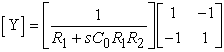(1)

for the circuit of Figure 1(a) and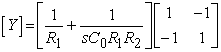(2)

for the circuit of Figure 1(b).

Thus, the circuit of Figure 1(a) simulates a floating series-RL impedance with equivalent resistance Req = R1 and equivalent inductance Leq = C0R1R2, while the circuit of Figure 1(b) simulates a parallel–RL admittance with Req = R1 and Leq = C0R1R2. In both the circuits, the value of Leq is controllable independently of the associated resistive part by a single variable resistance R2.Download asVeiw figureFigures index
Figure 1. The canonic floating inductance simulators (a) series-RL FI simulator (b) parallel-RL FI simulator

From equations (1) and (2), it can be readily deduced that the various sensitivity coefficients of the realized equivalent inductance and resistance with respect to passive elements would be in range of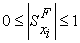(3)

where F represents Leq or Req and xi represents any of R1, R2 and C0 and the circuits, thus, enjoy low sensitivity properties.

### 3. The Effect of Non-ideal Parameters of the CFOAs

Considering the various non-ideal parasitic impedances of the CFOAs, namely, the finite input impedance looking into terminal-X as RX, the output impedance looking into terminal-Z (ZP) consisting of a parasitic resistance RP in parallel with a parasitic capacitance CP and the input impedance looking into Y-terminal (ZY) consisting of a parasitic resistance RY in parallel with a parasitic capacitance CY, the non-ideal Y-parameters of the two circuits are found to be:

For the circuit of Figure 1(a)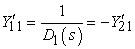(4)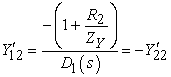(5)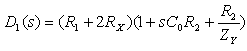(6)

For the circuit of Figure 1(b)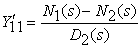(7)

where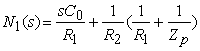(8)

and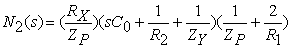(9)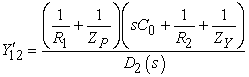(10)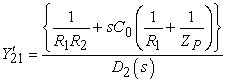(11)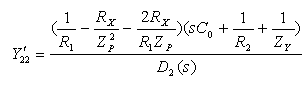(12)

where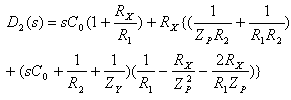(13)

Note that if we take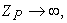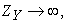and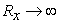, the non-ideal Y-parameters approach their ideal values as given in equations (1) and (2). From the non-ideal Y-parameters, it is clear that like all other FI circuits, the performance of both the circuits will depart from its ideal intended one, at high frequencies.

A comparison of the various features of the proposed circuits with CFOA-based and CCII-based (realizable with CFOAs) loss-less/lossy FI circuits known earlier has been carried out in Table 1. In making this table, it is taken into account that a CCII+ is realizable with one CFOA whereas a CCII- can be realized with two CFOAs).

#### Table 1. Comparison with the Earlier Known CircuitsDownload asPowerPoint Slide

Veiw figure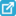View current table in a new window

From Table 1, it is clear that the circuits presented in this paper are the only ones which possess the following properties simultaneously, namely, (i) employment of a canonical number of passive components (ii) employing only two CFOAs (iii) complete absence of any component-matching requirements and (iv) single-resistor-tunability of the realized FIs.

### 4. Experimental and Simulation Results

The validity of the proposed FI simulators has been verified by implementing them with commercially available AD844-type CFOAs and 5% tolerance RC elements, as well as by SPICE simulations based upon a macromodel of AD844.Download asVeiw figureFigures index
Figure 2. Frequency response of the BPF realized from the proposed FI of Fig.1 (a)

The workability of the simulated FI configuration of Figure 1(a) has been verified by employing it in the realization of a tunable band pass filter (BPF), by connecting a capacitor C1 in series with its port-1 (or port -2) of the simulated inductor, with a resistor RL connected at its port 2 (or port 1) and then taking the output as the voltage across RL. The component values chosen were: (i) Set-I: R1 = 1k = R2 = RL, C1 = 1nF = C0 and (ii) Set-II: R1 = RL = 1k, R2 = 4.7k, C1= 1nF = C0 chosen to provide theoretical values of f0 as 159 kHz and 73.34 kHz respectively. The experimental results of these designs have been shown in Figure 2 wherein the experimental values of f0 have been found to be 159 kHz and 74 kHz respectively. The experimental results of Figure 2, thus, confirm the workability as well as the tunability of the inductance value (and hence, f0) with R2.

The workability of the parallel- RL FI of Figure 1(b) has been confirmed by using it in the design of a 4th order Butterworth filter based upon the normalized passive RLC prototype of Figure 3(a) and using transformation T-2{3} from [25, 26]. This transformation scales all the impedances of the RLC prototype of Figure 3(a) by a frequency-dependent scaling factor F(s) =1/ (1+s) which transforms a resistor into a parallel RC, an inductor into a parallel RL and a capacitor into a parallel combination of a capacitor and a FDNR (frequency-dependent-negative-resistance; an element having impedance of type Z(s) =1/Ds2), as shown in Figure 3(b). Note that in the transformed circuit of Figure 3(b), the floating parallel RL simulator of Figure 1(b) can be employed directly in place of both the parallel-RL branches. Furthermore, the two shunt CD-branches can also be simulated from the RC: CR transformed version of the circuit of Figure 1(b) with anyone of its two ports grounded. However, such a circuit would require two CFOAs for each grounded shunt parallel CD branch and would not be economical. Hence, to reduce the total component count, instead of using the suggested two- CFOA-based circuit, we have used a simpler one-CFOA-based circuit shown in Figure 3(c) to simulate both the shunt-CD branches encountered in the transformed prototype of Figure 3(b). The final circuit, thus, obtained has been shown in Figure 3 (d).

This circuit enables direct incorporation of the lossy FI of Figure 1(b) and grounded CD branch realized with a single CFOA circuit of Figure 3(c) into the design where the component values, as shown, have been obtained for a de-normalized cut-off frequency of f0 = 100kHz.

The SPICE simulations have revealed the cut-off frequency as 98 kHz which is quite close to the theoretical value of 100 kHz. The SPICE-generated frequency response of the circuit is shown in Figure 3(e). These simulation results, thus, confirm the workability of the FI circuit of Figure 1(b).Download asVeiw figureFigures index
Figure 3. Application and SPICE simulation results of the FI circuit of Figure 1(b): (a) Normalized 4th-order Butterworth Low Pass Filter; (b) Filter obtained by applying Senani’s transformation T-2 from [25,26] on the circuit of Figure 3(a); (c) An exemplary realization of a grounded parallel CD branch; (d) Final 4th- order Butterworth active filter obtained by replacing various RL and CD immittances of Figure 3(b) by the circuits of Figure 1(b) and Fig 3 (c); (e) SPICE generated Frequency response of the 4th-order Butterworth Low Pass Filter of Figure 3(d)

### 5. Discussions

Note that, in case of the BPF responses of Figure 2 while SPICE simulations take all the passive component values to be exact, in hardware implementation, RC components used were having 5% tolerances (hence, were not exact). As a consequence, the deviation of the practical responses from that exhibited by SPICE simulations is attributed to the passive component tolerances.

Further, it must be mentioned that the circuit of Figure 3(d) should not be taken as the recommended best method to design a 4th order Butterworth filter using CFOAs. This particular method has been applied here only as a vehicle to demonstrate the use of the FI of Figure 1(b) and to check its workability in higher order filter designs.

### 6. Concluding Remarks

Two canonic synthetic floating inductors have been discussed which, like the recently proposed FI of , use only two CFOAs, however, in contrast to the earlier circuit of , which requires two matched capacitors, three resistors (two of which are also required to be identical) and a cancellation constraint (for realizing a lossless FI), the discussed circuits provide the following advantageous features which are not available simultaneously either in the FI circuit of  or any other CC/CFOA based FIs known earlier: (i) use of a canonical number of passive components namely, only a single capacitor and two resistors (ii) employment of only two CFOAs for realizing an FI (iii) realization of the intended type of FIs without requiring any equality constraints or cancellation conditions, and (iv) the availability of single-resistance-tunability of the realized equivalent inductance value in both the cases.

The workability of the discussed FI circuits has been confirmed by experimental results and SPICE simulations based upon AD844-type CFOAs.

### Acknowledgement

The authors wish to thank Dr. Dinesh Prasad and Dr. R. K. Sharma for their help in the preparation of this manuscript. Thanks are also due to the anonymous reviewers for their constructive suggestions and feedback.

### Notes

1. Although reference  shows that three passive elements and a single modified CFOA are sufficient to realize a variety of grounded impedances but the so-called modified CFOA is, in fact, actively realized from a composite connection of a CCII+ and a CCII- and therefore, would call for at least three CFOAs of the normal kind (one for CCII+ and two for the CCII-).

2. The circuit of Figure 1(a) was briefly presented in a conference  and before that, had been mentioned in , as an unpublished circuit. However, the circuit of Figure 1(b) is completely new.

3. For further details of the various transformations, see [25, 26]

### References

  Senani, R., “Novel active-RC circuit for floating inductor simulation,” IEE Electron Lett, 15(21), 679-680, Oct. 1979.In article View Article  Senani, R., “New tunable synthetic floating inductors,” IEE Electron Lett, 16(10), 382-383, May 1980.In article View Article  Senani, R., “Floating ideal FDNR using only two current conveyors,” IEE Electron Lett, 20(5), 205-206 March 1984.In article View Article  Senani, R., “On the realization of floating active elements,” IEEE Trans Circuits Syst., 33(3), pp. 323-324, March 1986.In article  Fabre, A., “Gyrator implementation from commercially available transimpedance operational amplifier,” IEE Electron Lett, 28(3), 263-264, Jan. 1992.In article View Article  Senani, R., “Realization of a class of analog signal processing/generation circuits: Novel configurations using Current feedback op-amps,” Frequenz, 52(9-10), 196-206, Sept.–Oct. 1998.In article View Article  Liu, S.I., and Hwang, Y.S., “Realization of R-Land C-D impedances using current feedback amplifier and its applications,” IEE Electron Lett, 30(5), 380-381, March 1994.In article View Article  Yuce, E., “Novel lossless and lossy grounded inductor simulators consisting of a canonical number of components,” Analog Integr Circuits Signal Process, 59(1), 77- 82, April 2009.In article View Article  Abuelma’atti, M.T., “Comment on “Novel lossless and lossy grounded inductor simulator consisting of canonical number of components,” Analog Integr Circuits Signal Process, 68(1), 139-141, July 2011.In article View Article  Yuce, E., “Reply to comment on “Novel lossless and lossy grounded inductor simulator consisting of canonical number of components,” Analog Integr Circuits Signal Process, 72(2), 505-507, Aug. 2012.In article View Article  F. Kacar and H. Kuntman, ‘CFOA-based lossless and lossy inductance simulators,’ Radioengineering, vol. 20, no. 3, pp. 627-631, Sept. 2011.In article  Abuelma’atti, M.T., “New grounded immittance function simulators using single current feedback operational amplifier,” Analog Integr Circuits Signal Process, 71(1), 95-100, April 2012.In article View Article  Lahiri, A. and Gupta, M., “Realizations of grounded negative capacitance using CFOAs,” Circuits Syst Signal Process, 30(1), 143-155, Feb. 2011.In article View Article  Yuce, E. and Minaei, S., “On the realization of simulated inductors with reduced parasitic impedance effects,” Circuits Syst Signal Process, 28(3), 451-465 June 2009.In article View Article  Chang, C.M. and Hwang, C.S., “Comment on Voltage-mode notch, low pass and band pass filter using current-feedback amplifier,” IEE Electron. Lett, 31(4), 246, Feb. 1995.In article View Article  Chang, C.M., Hwang, C.S. and Tu, S. H., “Voltage-mode notch, low pass and band pass filter using current-feedback amplifiers,” IEE Electron Lett, 30(24), 2022-2023, Nov. 1994.In article View Article  Newcomb, R. W., Active Integrated Circuit Synthesis, Prentice-Hall, Inc. Englewood Cliffs, New Jersey, 1968, 151.In article  Bhushan, M. and Newcomb, R. W., “Grounding of capacitors in integrated circuits,” IEE Electron. Lett, 3(4), 148-149, April 1967.In article View Article  Psychalinos, C., Pal, K. and Vlassis, S., “A floating generalized impedance converter with current feedback operational amplifiers,” Int J Electron Commun (AEU), 62(2), 81-85, Feb. 2008.In article View Article  Senani, R., Bhaskar, D. R., Gupta, S. S. and Singh, V. K., “A configuration for realizing floating, linear, voltage-controlled resistance, inductance and FDNC elements,” Int J Circuit Theory Appl, 37(5), 709-719, June 2009.In article View Article  Senani, R., Bhaskar, D. R., Singh, A. K. and Singh, V. K., Current feedback operational amplifiers and their applications, Springer, USA, 2013, Chapter 3 Simulation of Inductors and other types of Impedances using CFOAs.In article  Senani, R., “Novel lossless synthetic floating inductor employing a grounded capacitor,” IEE Electron Lett, 18(10), 413-414, May 1982; also see Erratum, ibid, August 1982 issue.In article  Senani, R. and Bhaskar, D. R., “New loss-less/lossy Synthetic floating inductance configuration realized with only two CFOAs,” Analog Integr Circuits Signal Process, 73(3), 981-987, Dec. 2012.In article View Article  Yuce, E. and Minaei, S., “A modified CFOA and its applications to simulated inductors, capacitance multipliers, and analog filters,” IEEE Trans Circuits Syst I, 55(1), 266-275, Feb. 2008.In article View Article  Senani, R., “Novel higher order active filter design using Current Conveyors,” IEE Electron Lett, 21(22), 1055-1057, Oct. 1985.In article View Article  Senani, R., “Network transformations for incorporating non-ideal simulated immittances in the design of active filters and oscillators,” IEE Proc. Pt. G, 134(4), 158-166, Aug. 1987.In article  Bhaskar, D. R. and Senani, R., “Simulation of a floating inductance: A new two-CFOA-based configuration,” Fifth Int. Conf. on Computational Intelligence, Modeling and Simulation (CIMSim), 2013, South Korea, 24-25 Sept. 2013, 381-383, Seoul.In article View Article  Ferri, G. and Guerrini, N., “High-valued passive element simulation using low-voltage low-power current conveyors for fully integrated applications,” IEEE Trans Circuits Syst II, 48(4), 405-409, April, 2001.In article View Article  Metin, B. and Cicekoglu, O., “A novel floating lossy inductance realization topology with NICs using current conveyors,” IEEE Trans Circuits Syst II, 53(6), 483-486, June 2006.In article View Article  Maundy, B., Gift, S. and Aronhime, P., “A novel hybrid active inductor,” IEEE Trans Circuits Syst II, 54(8), 663-667, Aug, 2001.In article View Article  Ferri, G., Guerrini, N., Silverii, E. and Tatone, A., “Vibration damping using CCII-based inductance simulators,” IEEE Trans Instrument Measurement, 57(5), 907-914, May 2008.In article View Article  Yuce, E., “Grounded inductor simulators with improved low-frequency performance,” IEEE Trans Instrument Measurement, 57(5), 1079-1084, May 2008.In article View Article  Senani, R., Bhaskar, D. R., Singh, A. K. and Singh, V. K., Current feedback operational amplifiers and their applications, Springer Science + Business Media, New York 2013, Ch. 3, 67.In article